# 16. The Fibonacci RetracementsThe topic of Fibonacci retracements is quite intriguing. To fully understand and appreciate the concept of Fibonacci retracements, one must understand the Fibonacci series. The origins of the Fibonacci series can be traced back to the ancient Indian mathematic scripts, with some claims dating back to 200 BC. However, in the 12th century, Leonardo Pisano Bogollo, an Italian mathematician from Pisa, known to his friends as Fibonacci discovered Fibonacci numbers.

The Fibonacci series is a sequence of numbers starting from zero arranged so that the value of any number in the series is the sum of the previous two numbers.

The Fibonacci sequence is as follows:

0 , 1, 1, 2, 3, 5, 8, 13, 21, 34,  55, 89, 144, 233, 377, 610…

Notice the following:
233 = 144 + 89
144 = 89 + 55
89 = 55 +34

Needless to say, the series extends to infinity. There are few interesting properties of the Fibonacci series.

Divide any number in the series by the previous number; the ratio is always approximately 1.618.

For example:
610/377 = 1.618
377/233 = 1.618
233/144 = 1.618

The ratio of 1.618 is considered as the Golden Ratio, also referred to as the Phi. Fibonacci numbers have their connection to nature. The ratio can be found in the human face, flower petals, animal bodies, fruits, vegetables, rock formation, galaxy formations etc. Of course, let us not get into this discussion as we would be digressing from the main topic.  For those interested, I would suggest you search on the internet for golden ratio examples, and you will be pleasantly surprised. Further into the ratio properties, one can find remarkable consistency when a number is in the Fibonacci series is divided by its immediate succeeding number.

For example:
89/144 = 0.618
144/233 = 0.618
377/610 = 0.618

At this stage, do bear in mind that 0.618, when expressed in percentage is 61.8%.

Similar consistency can be found when any number in the Fibonacci series is divided by a number two places higher.

For example:
13/34 = 0.382
21/55 = 0.382
34/89 = 0.382

0.382, when expressed in percentage terms, is 38.2%

Also, consistency is when a number in the Fibonacci series is divided by a number 3 place higher.

For example:
13/55 = 0.236
21/89 = 0.236
34/144 = 0.236
55/233 = 0.236

0.236, when expressed in percentage terms, is 23.6%.

## 16.1 – Relevance to stocks markets

It is believed that the Fibonacci ratios, i.e. 61.8%, 38.2%, and 23.6%, finds its application in stock charts. Fibonacci analysis can be applied when there is a noticeable up-move or down-move in prices.  Whenever the stock moves either upwards or downwards sharply, it usually tends to retrace back before its next move. For example, if the stock has run up from Rs.50 to Rs.100, it is likely to retrace back to probably Rs.70 before moving Rs.120.

‘The retracement level forecast’ is a technique that can identify upto which level retracement can happen. These retracement levels provide a good opportunity for the traders to enter new positions in the trend direction.  The Fibonacci ratios, i.e. 61.8%, 38.2%, and 23.6%, help the trader identify the retracement’s possible extent. The trader can use these levels to position himself for trade.

Have a look at the chart below: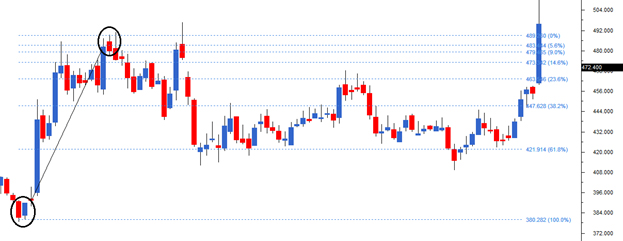I’ve encircled two points on the chart, at Rs.380 where the stock started its rally and at Rs.489, where the stock prices peaked.

I would now define the move of 109 (380 – 489) as the Fibonacci upmove.  As per the Fibonacci retracement theory, after the upmove one can anticipate a correction in the stock to last up to the Fibonacci ratios. For example, the first level up to which the stock can correct could be 23.6%. If this stock continues to correct further, the trader can watch out for the 38.2% and 61.8% levels.

Notice in the example shown below, the stock had retraced up to 61.8%, which coincides with 421.9, before it resumed the rally.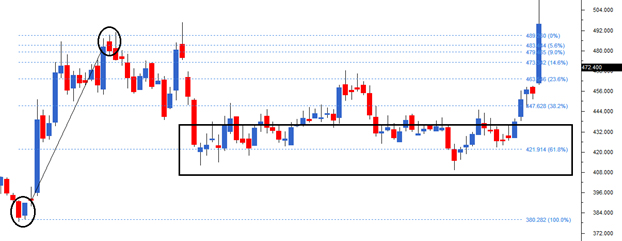We can arrive at 421 by using simple math as well –

Total Fibonacci up move = 109

61.8% of Fibonacci up move = 61.8% * 109 = 67.36

Retracement @ 61.8% = 489- 67.36 = 421.6

Likewise, we can calculate for 38.2% and the other ratios. However one need not manually do this as the software will do this for us.

Here is another example where the chart has rallied from Rs.288 to Rs.338. Therefore 50 points move makes up for the Fibonacci upmove. The stock retraced back 38.2% to Rs.319 before resuming its up move.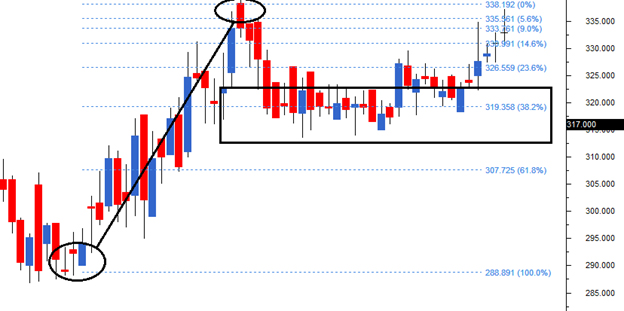The Fibonacci retracements can also be applied to falling stocks to identify levels upto which the stock can bounce back. In the chart below (DLF Limited), the stock started to decline from Rs.187 to Rs. 120.6 thus making 67 points as the Fibonacci down move.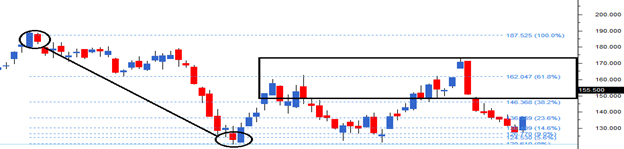After the down move, the stock attempted to bounce back retracing back to Rs.162, which is the 61.8% Fibonacci retracement level.

## 16.2 – Fibonacci Retracement construction

As we now know, Fibonacci retracements are movements in the chart that go against the trend. To use the Fibonacci retracements, we should first identify the 100% Fibonacci move. The 100% move can be an upward rally or a downward rally. To mark the 100% move, we need to pick the most recent peak and trough on the chart. Once this is identified, we connect them using a Fibonacci retracement tool. This is available in most of the technical analysis software packages including Zerodha’s Pi 🙂

Here is a step by step guide:

Step 1) Identify immediate peak and trough. In this case, the trough is at 150, and the peak is at 240. The 90 point moves make it 100%.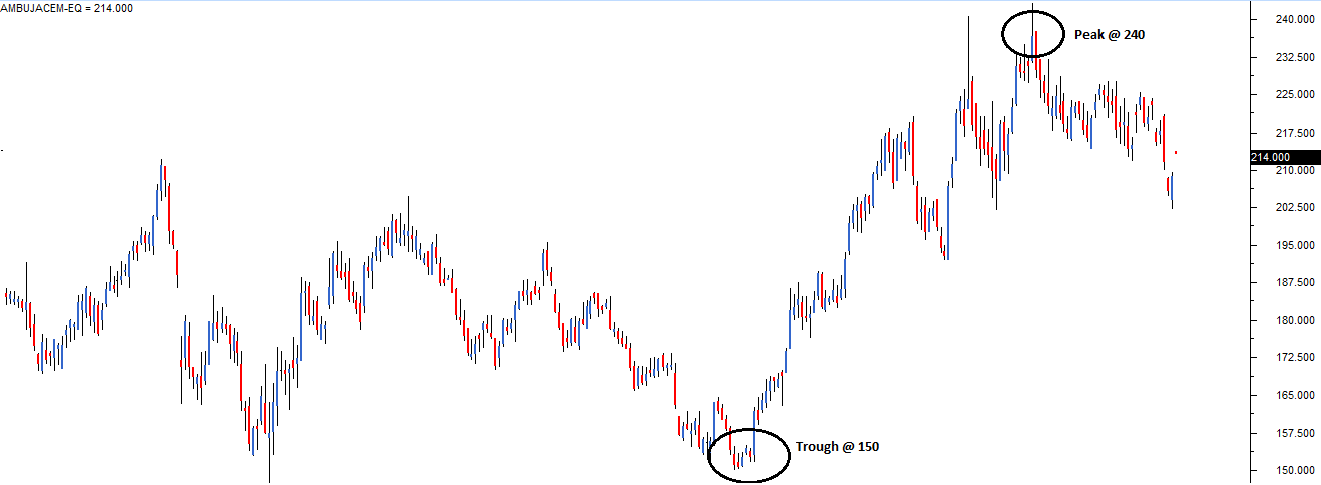Step 2) Select the Fibonacci retracement tool from the chart tools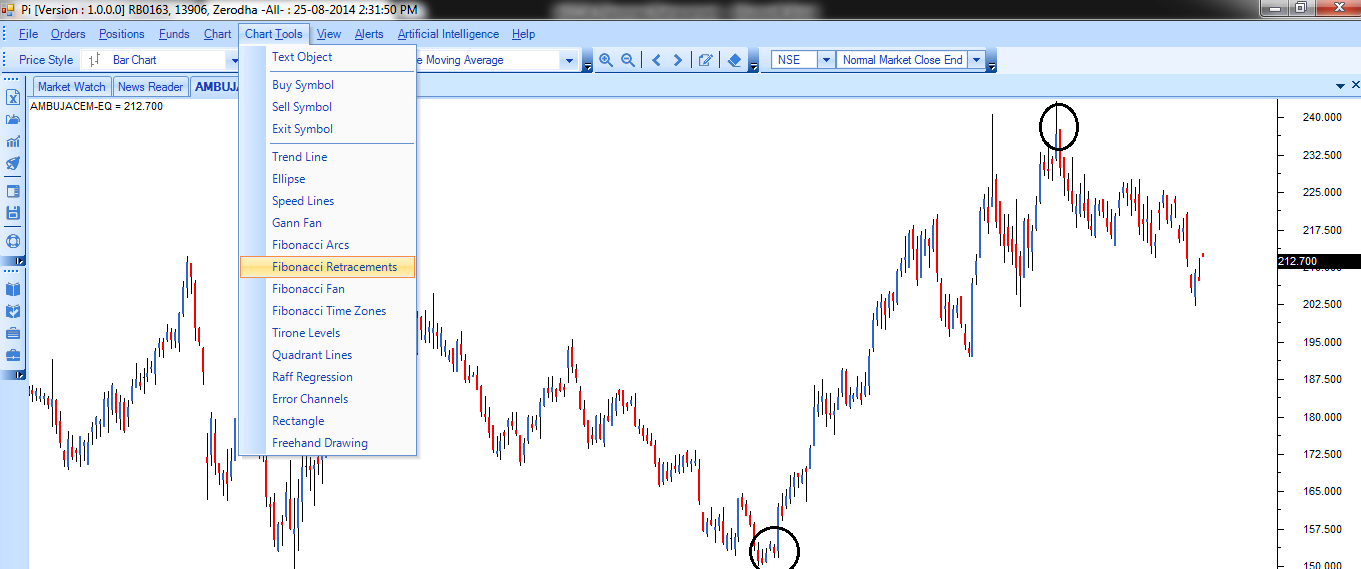Step 3) Use the Fibonacci retracement tool to connect the trough and the peak.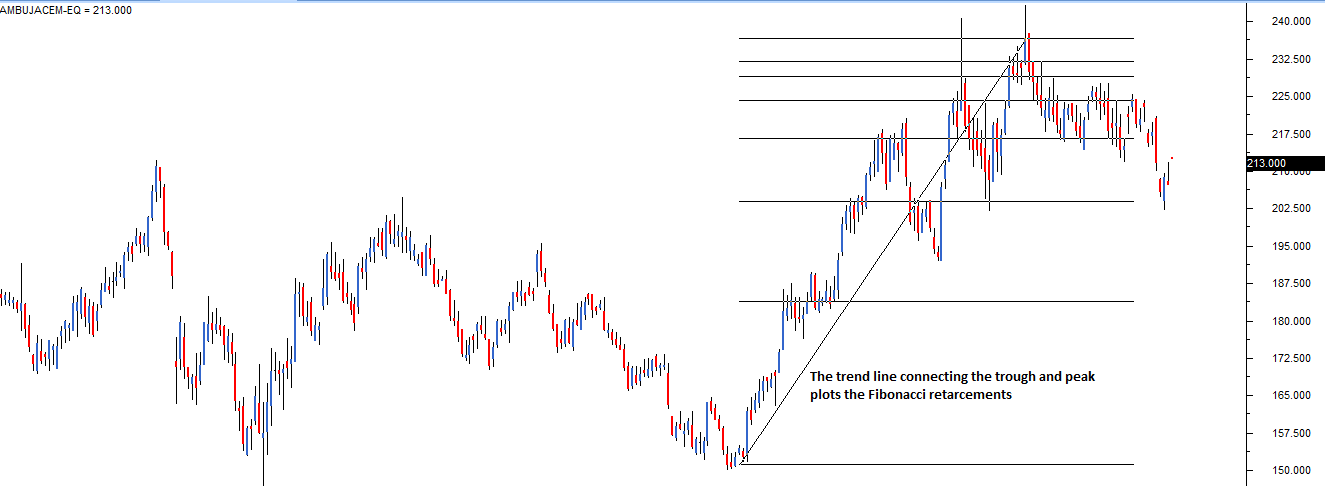After selecting the Fibonacci retracement tool from the charts tool, the trader has to click on trough first, and without un-clicking, he has to drag the line till the peak. While doing this, simultaneously, the Fibonacci retracements levels start getting plotted on the chart. However, the software completes the retracement identification process only after selecting both the trough and the peak. This is how the chart looks after selecting both points.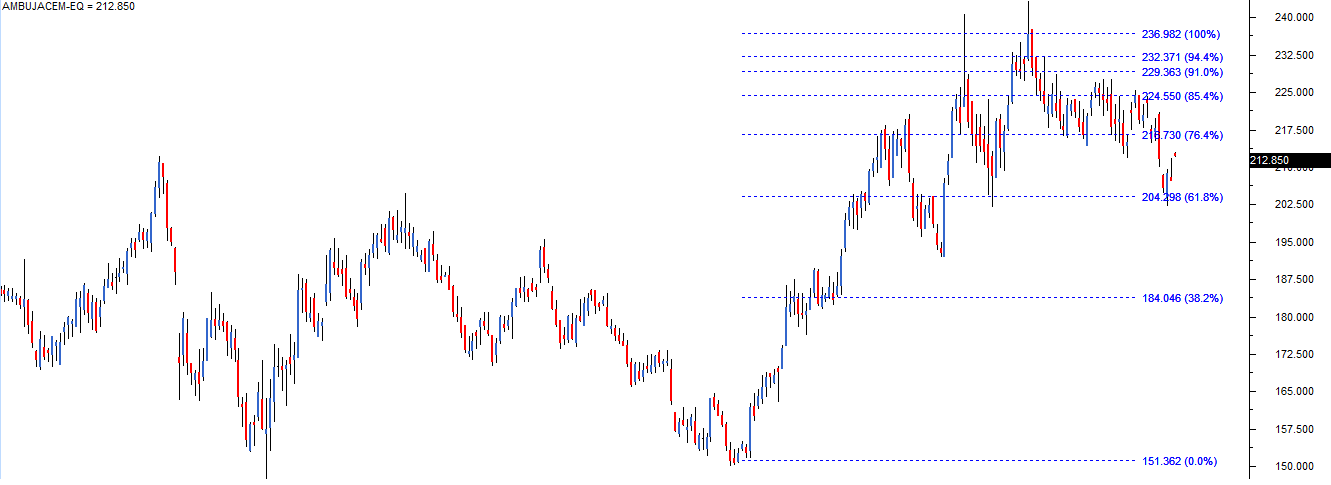You can now see the Fibonacci retracement levels are calculated and loaded on the chart. Use this information to position yourself in the market.

## 16.3 – How should you use the Fibonacci retracement levels?

Think of a situation where you wanted to buy a particular stock, but you have not been able to do so because of a sharp run-up in the stock. The most prudent action to take would be to wait for a retracement in the stock in such a situation. Fibonacci retracement levels such as 61.8%, 38.2%, and 23.6% act as a potential level upto which a stock can correct.

By plotting the Fibonacci retracement levels, the trader can identify these retracement levels, and therefore position himself for an opportunity to enter the trade. However please note like any indicator, use the Fibonacci retracement as a confirmation tool.

I would buy a stock only after it has passed the other checklist items. In other words, my conviction to buy would be higher if the stock has:

1. Formed a recognizable candlestick pattern
2. The stoploss coincides with the S&R level.
3. Volumes are above average.

Along with the above points, if the stoploss also coincides with the Fibonacci level, I know the trade setup is well aligned to all the variables, and hence I would go in for a strong buy. The word ‘strong’ usage indicates the level of conviction in the trade set up. The more confirming factors we use to study the trend and reversal, more robust is the signal. The same logic can also be applied for the short trade.

### Key takeaways from this chapter

1. The Fibonacci series forms the basis for Fibonacci retracement
2. A Fibonacci series has many mathematical properties. These mathematical properties are prevalent in many aspects of nature.
3. Traders believe the Fibonacci series has its application in stock charts as it identified potential retracement levels.
4. Fibonacci retracements are levels (61.8%, 38.2%, and 23.6% ) upto which a stock can retrace before it resumes the original directional move.
5. At the Fibonacci retracement level, the trader can look at initiating a new trade. However, before initiating the trade, other points in the checklist should also confirm.

1.ashok says:

now a days rather than fibonacci levels what i have observed is retracement of 33, 42 to 45, 52 and 65 to 68 percent range. just accidental or what i want to know. to be precise i dont have data to give but i hope ypu have them to check and reply.

•Karthik Rangappa says:

Without actual data this would be hard to verify 🙂

•Shriram Sarnaik says:

D/sir
I am zerodha customer and make my trade through kite by Android phone only. Is there is application on chart for Fibbonacci retractmen

•Karthik Rangappa says:

Retracement is available on Kite, request you to give it another look. Thanks.

•PRASHANTH av says:

Don’t think it’s available on kite

•Aditya Bhat says:

Its available.
1.open any chart
2. click on draw (which is available after cross hair and info. has pencil icon )
3. in select tool select Fibonacci.
4. click latest lowest point on the chart and move the cursor to highest high

•shreyas says:

FIBONACCI EXTENSION DRAWING TOOL ISNT AVAILABLE ON ZERODHA. KINDLY MAKE IT AVAILABLE.

•Karthik Rangappa says:

Its available, Shreyas. Please check the studies.

•Suraj says:

Sir, I am unable to draw the Fibonacci levels from mobile at kite app. If there are any tutorial videos regarding drawing of Fibonacci. Would be helpful for me.

•Karthik Rangappa says:

What is the problem that you are facing, Suraj?. Maybe you should call our support executive, they will help you with it.

•SANTANU HALDER says:

The concept of fivonachhi is not available in Zerodha Kite platform. Kindly intimate how can I apply the fivonachhi retracement in zerodha kite.

SANTANU HALDER
USER ID : EO3455

•Karthik Rangappa says:

Santanu, I’d suggest you speak to our customer care desk for this, they will help you with it.

2.ashok says:

in nest chart spot there is premarket values inclueded which distorts values. can you set right the problem so that i can give data with dates.
for eg. on 10 nov 8264 is low but nse site gives 8304 low for that day.the reason being values of 9 to 9.15 taken

•Hanan Delvi says:

Yeah, Ashok. I’ve seen this distortion that you mention. Thanks for reporting it. We’ve sent this over to our developers and hope to have it fixed within the next few weeks. 🙂

•Mayank Samar says:

hanan, can u make a video on fibonachi and mail that will be much better to understand ,

•Karthik Rangappa says:

Will pass that message to him 🙂

3.Harshad Salvi says:

It is very important that we identify the reasonable upmove or downmove. The moot question is what constitutes this reasonable / significant move? What should the bare minimum move in terms of % with respect price of the particular scrip?

•Karthik Rangappa says:

Are you referring to the prior trend up move or down move? If yes, I usually like to look at last 5 days trend…I consider a move over and above 5-8% as reasonable.

•Harshad Salvi says:

Is it fair to look at the prior up/down move of only last 5 days ? In the examples given above also it seems the prior uptrend / downtrend extending to large no. of days or even weeks for that matter.
Also, what should be the signal that trend might get reversed? Or we must wait till we get the confirmed signal of reversal of trend?

•Karthik Rangappa says:

The larger the look back period, the better. However if I have to put a minimum number to it then it would be 5 days. I guess it pays off to wait for a confirmed signal which indicates the trend could be reversing.

4.Thiyagu says:

Is my understanding right on the below?

You said, “For example, the first level up to which the stock can correct would be 61.8%. If this stock continues to correct further, the trader can watch out for the 38.2% level. So on and so forth.”

In the above case, you said that the first level retracement is up to 61.8 and then look for 38.2 and so on. So, if I calculate the 38.2 and 26.3 of the Fibonacci move, obviously it will be less than 61.8. Then, how come these will be the next levels. Correct me if I am wrong.

As I understand, the first retrace may be 23.6 and if it breaks we can wait for 38.2 and so on.

I am trading options, can I use last 9 trading days data of nifty or bank nifty to identify the next retracements? or suggest me with suitable days. I might hold the position for 2 days to 1 week, which will be best?

Thank you

•Karthik Rangappa says:

Thiyagu – You are absolutely correct, an obvious typo error. Thanks for pointing this, will make the corrections right away.
For short term trading, I personally prefer 9 or 21 day data points.

5.Girish Vyas says:

Gann cycle work perfectly then why people use other failed methods . i request zerodha to literate traders about Gann cycle method